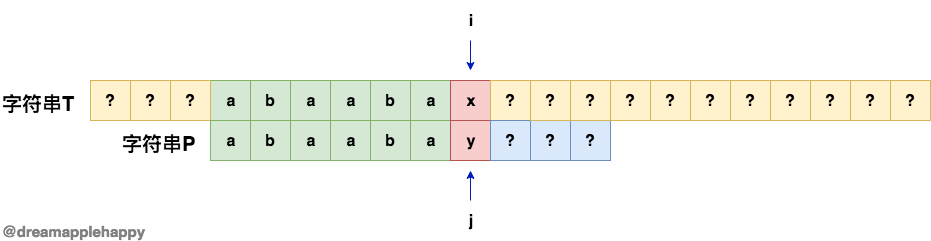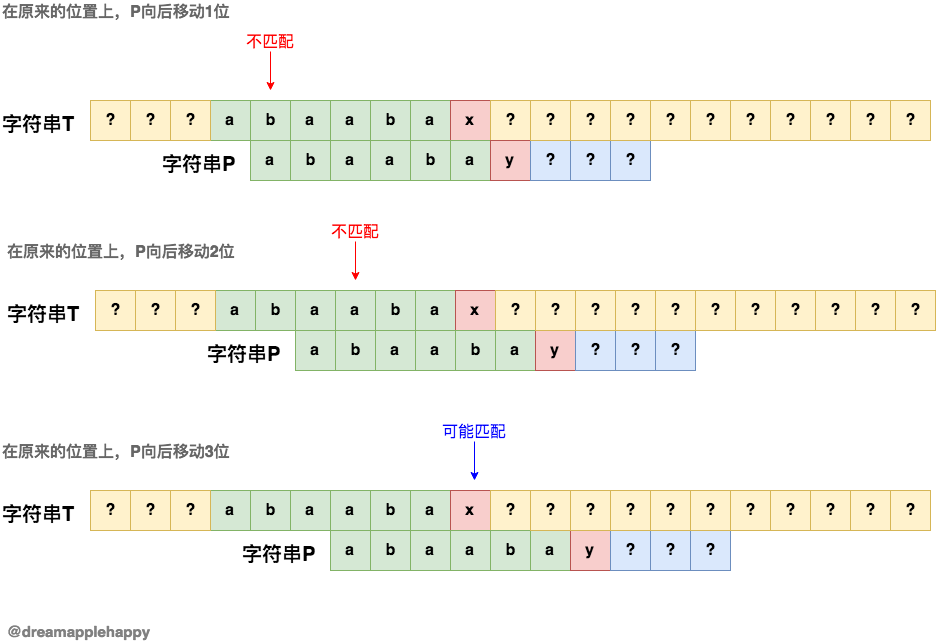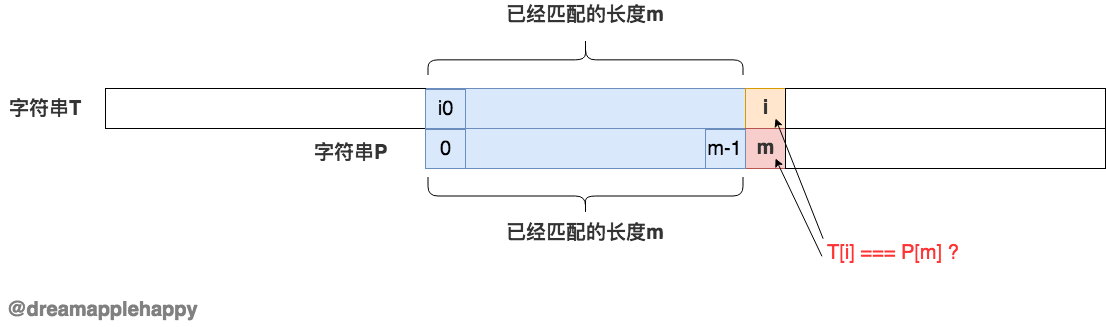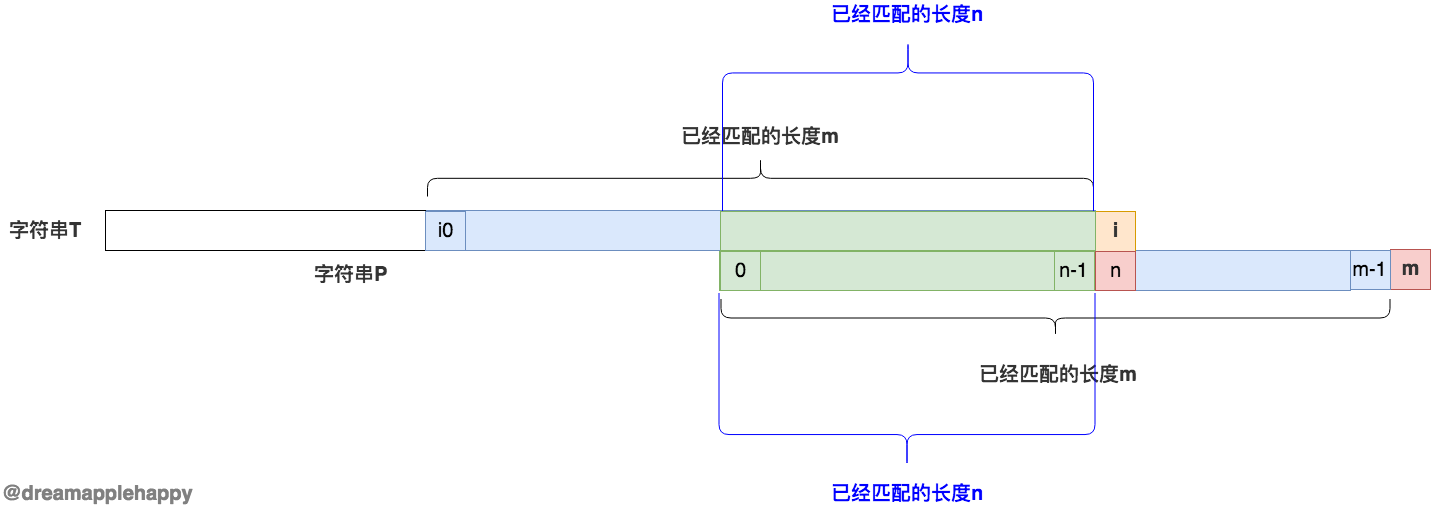``````有两个字符串T和P；需要我们从T中找出与P字符串匹配的下标的位置
``````

``````// JavaScript 版本代码
function searchPatternIndex(T, P) {
// 计算T和P的长度
const tLen = T.length;
const pLen = P.length;

// 要查找的位置，默认是0
let i0 = 0;

// T和P字符串查找比对的位置
let i = 0;
let j = 0;

while (i < tLen) {
// T和P当前位置的字符相等
if (T[i] === P[j]) {
i++;
j++;
// 找到P的位置
if (j === pLen) {
return i0;
}
} else {
// 如果不相等的话，i0的位置向后移动一位
i0++;
// 从新的位置开始对比
i = i0;
// 再次从P字符串的开始进行比对
j = 0;
}
}
// 没有找到的话返回-1
return -1;
}

// 测试代码
console.log(searchPatternIndex("jkajsdiuuqiwenasdjhababanbajhsdyqbhjabduagd", "ababa")); // 19
````````````function computeMoveSteps(P) {
// 计算P的长度
const pLen = P.length;
// 不同匹配长度下需要移动的值
const f = [];
// 初始值f = 0
f = 0;

// 当前位置下已经匹配的字符串的最后一个字符的下标
let k0;
// 当前位置下，如果后面的字符不匹配；已经匹配的字符串需要向后移动的值
let kLen;

for (let k = 1; k < pLen; k++) {
// 获取最后一个字符的下标
k0 = k - 1;
kLen = f[k0];

while (P[k] !== P[kLen]) {
// k0是匹配长度是kLen情况下的最后一个字符的下标
k0 = kLen - 1;
// 终止条件
if (k0 < 0) {
break;
}
// 获取匹配长度是kLen情况下可以移动的数值
kLen = f[k0];
}

// 如果k0小于0 说明在一直没有找到匹配
if (k0 < 0) {
f[k] = 0;
} else {
// 说明在已经匹配了k0长度的情况下 找到了匹配
f[k] = f[k0] + 1;
}
}

return f;
}

// 测试
console.log(computeMoveSteps("abababa")); // [ 0, 0, 1, 2, 3, 4, 5 ]
``````

``````function findPatternIndex(T, P) {
// 计算T和P的长度
const tLen = T.length;
const pLen = P.length;
// 不同匹配长度下可以向后移动的位数数组
const f = computeMoveSteps(P); // computeMoveSteps函数上面已经有了
// T中匹配P的位置
let index = 0;
// 用i和j分别表示T和P要比较的字符的下标，初始值都为0
let i = 0;
let j = 0;
// P可以向后移动的位置
let step;

while(i < tLen) {
// 如果T[i]和P[j]的值相等，那么i和j都各自加1
if(T[i] === P[j]) {
i++;
j++;
// 如果j的值等于pLen那就说明已经找到了
if(j === pLen) {
return index;
}
} else {
// 如果j等于0 那么说明刚开始就不匹配
if(j === 0) {
// index的值加1
index++;
// 重新初始化i和j的值
i = index;
j = 0;
} else {
// 这个时候我们有可能直接将j向后移动几个位置
step = f[j-1];
j = step;
index = i - step;
}
}
}

// 如果中途没有返回值，那么就是没有找到，返回-1
return -1;
}

// 测试